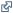You are here:

# Texas Photochemical Modeling Results—Model Performance Statistics by Area and Day

Interactive bar chart and data table of model performance statistics comparing the results of the TCEQ’s air quality modeling with surface observations.

The purpose of evaluating model performance is to determine how well the model replicates an historical episode. Performance statistics do not feed directly into future ozone estimates or regulatory decisions.

To compare results of ozone photochemical modeling to actual observed data from surface monitoring sites, the TCEQ calculates the following statistical metrics, recommended by the EPA (Section 3.2.1 of Modeling Guidance for Demonstrating Attainment of Air Quality Goals for Ozone, PM2.5, and Regional Haze, 2014 December):

• Mo - Mean of Observed Values
• Mm - Mean of Modeled Values (ideal if Mm = Mo)
• MB - Mean Bias (mean difference; ideal value = 0)
• ME - Mean Error (mean absolute difference; ideal value = 0)
• RMSE - Root Mean Squared Error (ideal value = 0)
• NMB - Normalized Mean Bias (relative mean difference; ideal value = 0)
• NME - Normalized Mean Error (relative mean absolute difference; ideal value = 0)
• FB - Fractional Bias (value range: -200% to 200%; ideal value = 0)
• FE - Fractional Error (value range: 0 to 200%; ideal value = 0)
• R2 - Coefficient of Determination (square of correlation coefficient; value range: 0 to 1; ideal value = 1)

This page shows interactive chart and data table of ozone performance statistics by days for a selected area from the 2006 photochemical modeling.

The statistical performance of the TCEQ's base case ozone modeling is comparable to and, in many cases, above average of other ozone modeling efforts discussed in a research paper Compilation and Interpretation of Photochemical Model Performance Statistics Published between 2006 and 2012(Simon, et al, Atmospheric Environment 61:124–139, November 2012).

The median value, Interquartile Range (IQR), and value range of ozone statistics presented in this paper (Fig. 4) are available to overlay on the chart for MB, ME, RMSE, NMB, NME, and R2 of hourly, daily maximum of hourly, and daily maximum of eight-hour data.

If you have any questions regarding this interactive chart, please send an email to amda@tceq.texas.gov with the subject "Texas Photochemical Modeling Results - Model Performance Statistics by Area and Day".

show instructions
Episode
Date
Valid
Data
Mo
ppb
Mm
ppb
MB
ppb
ME
ppb
RMSE
ppb
NMB
%
NME% FB
%
FE
%
R2

select an episode